28 May 2023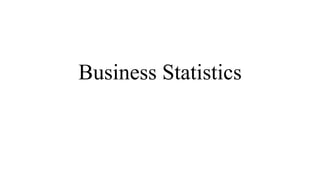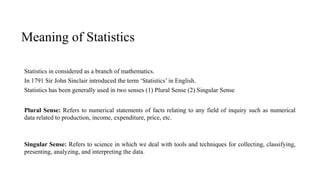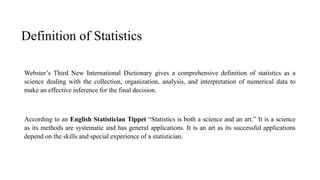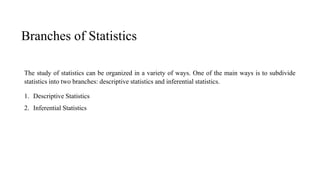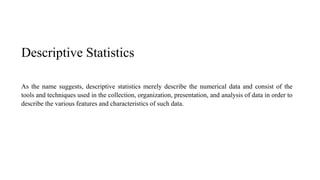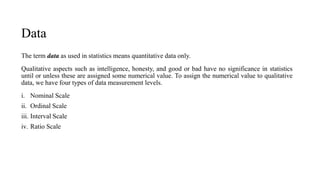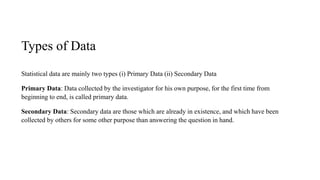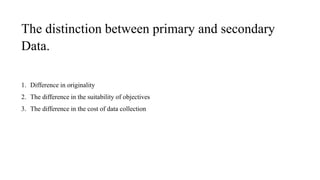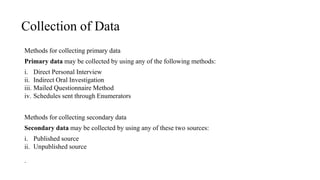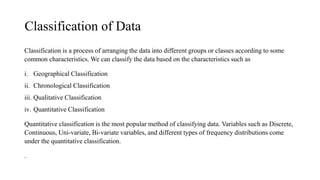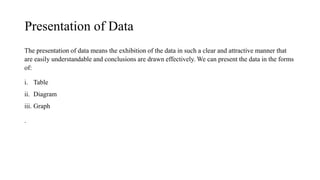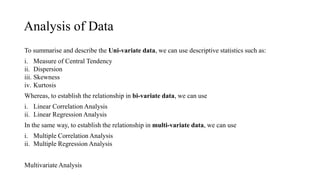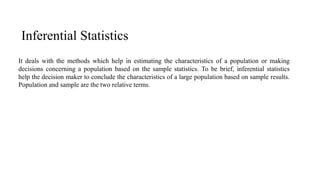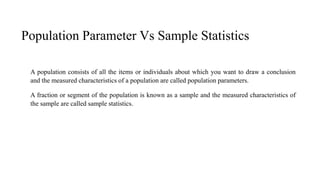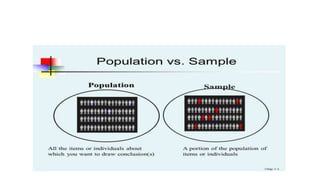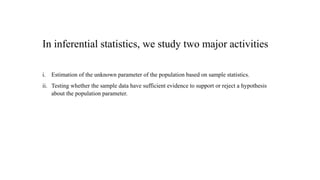1 sur 17

• 2. Meaning of Statistics Statistics in considered as a branch of mathematics. In 1791 Sir John Sinclair introduced the term ‘Statistics’ in English. Statistics has been generally used in two senses (1) Plural Sense (2) Singular Sense Plural Sense: Refers to numerical statements of facts relating to any field of inquiry such as numerical data related to production, income, expenditure, price, etc. Singular Sense: Refers to science in which we deal with tools and techniques for collecting, classifying, presenting, analyzing, and interpreting the data.
• 3. Definition of Statistics Webster’s Third New International Dictionary gives a comprehensive definition of statistics as a science dealing with the collection, organization, analysis, and interpretation of numerical data to make an effective inference for the final decision. According to an English Statistician Tippet “Statistics is both a science and an art.” It is a science as its methods are systematic and has general applications. It is an art as its successful applications depend on the skills and special experience of a statistician.
• 4. Branches of Statistics The study of statistics can be organized in a variety of ways. One of the main ways is to subdivide statistics into two branches: descriptive statistics and inferential statistics. 1. Descriptive Statistics 2. Inferential Statistics
• 5. Descriptive Statistics As the name suggests, descriptive statistics merely describe the numerical data and consist of the tools and techniques used in the collection, organization, presentation, and analysis of data in order to describe the various features and characteristics of such data.
• 6. Data The term data as used in statistics means quantitative data only. Qualitative aspects such as intelligence, honesty, and good or bad have no significance in statistics until or unless these are assigned some numerical value. To assign the numerical value to qualitative data, we have four types of data measurement levels. i. Nominal Scale ii. Ordinal Scale iii. Interval Scale iv. Ratio Scale .
• 7. Types of Data Statistical data are mainly two types (i) Primary Data (ii) Secondary Data Primary Data: Data collected by the investigator for his own purpose, for the first time from beginning to end, is called primary data. Secondary Data: Secondary data are those which are already in existence, and which have been collected by others for some other purpose than answering the question in hand.
• 8. The distinction between primary and secondary Data. 1. Difference in originality 2. The difference in the suitability of objectives 3. The difference in the cost of data collection
• 9. Collection of Data Methods for collecting primary data Primary data may be collected by using any of the following methods: i. Direct Personal Interview ii. Indirect Oral Investigation iii. Mailed Questionnaire Method iv. Schedules sent through Enumerators Methods for collecting secondary data Secondary data may be collected by using any of these two sources: i. Published source ii. Unpublished source .
• 10. Classification of Data Classification is a process of arranging the data into different groups or classes according to some common characteristics. We can classify the data based on the characteristics such as i. Geographical Classification ii. Chronological Classification iii. Qualitative Classification iv. Quantitative Classification Quantitative classification is the most popular method of classifying data. Variables such as Discrete, Continuous, Uni-variate, Bi-variate variables, and different types of frequency distributions come under the quantitative classification. .
• 11. Presentation of Data The presentation of data means the exhibition of the data in such a clear and attractive manner that are easily understandable and conclusions are drawn effectively. We can present the data in the forms of: i. Table ii. Diagram iii. Graph .
• 12. Analysis of Data To summarise and describe the Uni-variate data, we can use descriptive statistics such as: i. Measure of Central Tendency ii. Dispersion iii. Skewness iv. Kurtosis Whereas, to establish the relationship in bi-variate data, we can use i. Linear Correlation Analysis ii. Linear Regression Analysis In the same way, to establish the relationship in multi-variate data, we can use i. Multiple Correlation Analysis ii. Multiple Regression Analysis Multivariate Analysis
• 13. Inferential Statistics It deals with the methods which help in estimating the characteristics of a population or making decisions concerning a population based on the sample statistics. To be brief, inferential statistics help the decision maker to conclude the characteristics of a large population based on sample results. Population and sample are the two relative terms.
• 14. Population Parameter Vs Sample Statistics A population consists of all the items or individuals about which you want to draw a conclusion and the measured characteristics of a population are called population parameters. A fraction or segment of the population is known as a sample and the measured characteristics of the sample are called sample statistics.
• 16. In inferential statistics, we study two major activities i. Estimation of the unknown parameter of the population based on sample statistics. ii. Testing whether the sample data have sufficient evidence to support or reject a hypothesis about the population parameter.